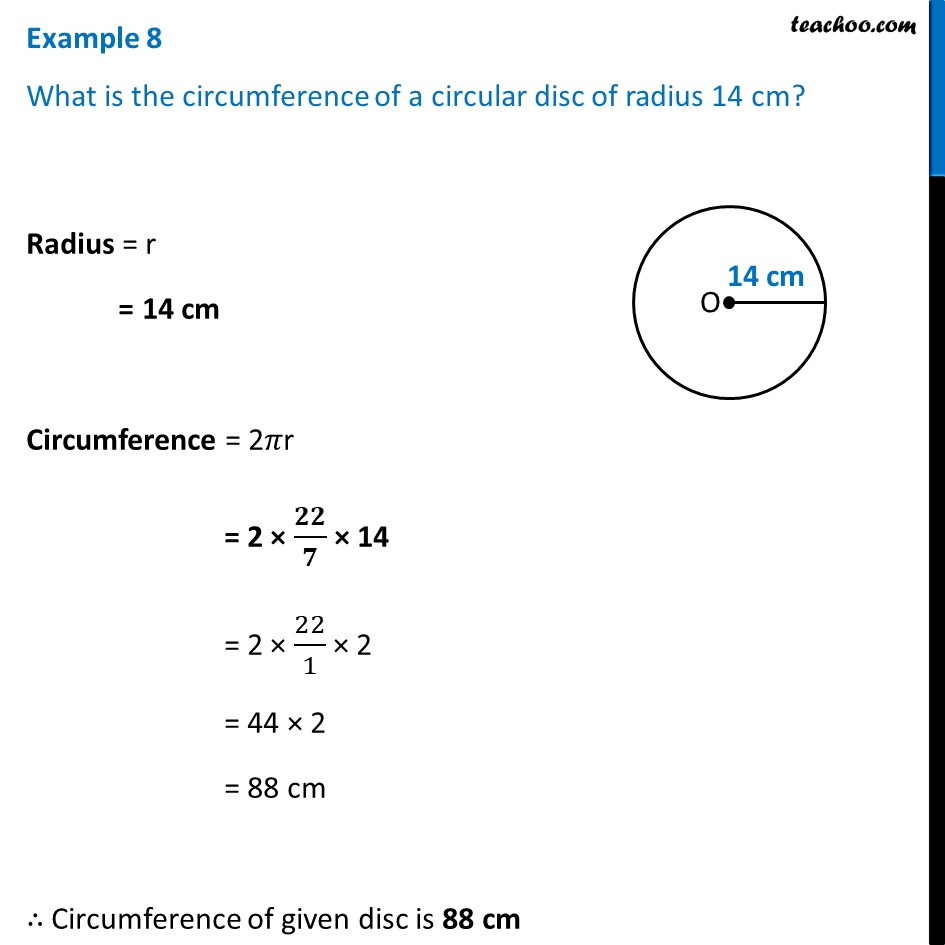Examples

Chapter 9 Class 7 Perimeter and Area
Serial order wiseLearn in your speed, with individual attention - Teachoo Maths 1-on-1 Class

### Transcript

Example 8 What is the circumference of a circular disc of radius 14 cm?Radius = r = 14 cm Circumference = 2𝜋r = 2 × 𝟐𝟐/𝟕 × 14 = 2 × 22/1 × 2 = 44 × 2 = 88 cm ∴ Circumference of given disc is 88 cm